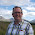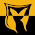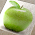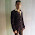## Wednesday, 27 July 2011

### EXCEL TIP: Eliminating #DIV/0! and other errors automatically using ISERROR

Excel is great for being able to apply the same calculation consistently by simply copying the formula to all of the relevant cells, however often there are times where some of the data isn't how we would like it and the formula throws up an error.

The most common of these is the #DIV/0! error that Excel returns when trying to divide a number by zero. As Excel struggles with the concept of infinity it returns this error. A common situation where this occurs is in a Gross Margin Percentage calculation. We may have multiple products with sales and profit figures and a formula to calculate the margin as a percentage, i.e. profit/sales formatted as a percentage.

This will work fine until you come to a product with no sales where the formula will return #DIV/0!.

This can be addressed using a combination of the IF statement and ISERROR (note that in Excel 2007 and 2010 there is a combined function called IFERROR, however the solution proposed here will also work in earlier versions of Excel). If you need a refresher on how the IF statement works take a look at :

If cell A1 contains the Sales figure and B1, the Profit, then to show the Margin Percentage in C1, we would format it as a percentage and use the following formula:

=B1/A1

This will return the #DIV/0! if cell A1 is empty or zero.

The ISERROR function returns TRUE if its argument returns an error and FALSE if not, therefore

=ISERROR(B1/A1)

will return TRUE when this #DIV/0! would occur.

We also need to decide what we want to appear instead of the error. In this scenario, I usually show 0% which is what I have assumed for the example.

Using the ISERROR formula above as the condition for the IF statement, we can return zero if it is an error, or the original calculation otherwise. So C1 becomes:

=IF(ISERROR(B1/A1),0,B1/A1)

No more #DIV/0! errors!

A similar approach can be used for any other common errors, such as when a looked up value is not in the list.

If you enjoyed this post, go to the top left corner of the blog, where you can subscribe for regular updates and your free report. If you wish to help me to provide future posts like this, please consider donating using the button in the right hand column.

1.I found this site following a link to the Index and Match tip. I have used index before, but looking forward to an apportunity to use Match. On this tip for ISERROR, I assume you have since found the IFERROR function that streamlines the If(ISERROR()) function. It uses the format of IFERROR(value,value_if_error). So you can say =iferror(a1/b1,0) and will return a 0 if a1/b1 produces and error.

1.But as he stated, the if(ISERROR()) format is used, the formula can be used in newer versions of Excel, but can also be used in Excel 97/2000. The IFERROR() format is not available in Excel 97/2000 so if used will eliminate any chance of a coworker using Excel 2000 being able to use your spreadsheet file on his computer, and not all companies are consistent enough to upgrade ALL computer system software versions at the same time. The last place I was employed only had Excel 2000 available on the computer I used, but most of the front office computers had Excel 2010 installed and in use. This made it so many Excel spreadsheets somebody in the front office would create intending for me to use it on the computer in my area wouldn't work at all on my computer, but any spreadsheet application I wrote would load and run successfully on any computer in the front office.

2.Thanks for providing these tips for solving Excel problems and working as data management expert in Excel. Get here best paper writing service for solving your assignments and past papers for your study. I hope it will help you for web content writing too.

3.i prefer to anticipate the error so as not to miss something else that may be lurking
in this case, i would avoid the #DIV/0! error by using:
=IF(A1,B1/A1,0)
then anything else that may be amiss will present itself
(it's also shorter and makes fewer function calls)

jim

1.That's a nice one for #DIV/0!, and certainly better from a technical point of view. IFERROR has more general application.

4.Just wondering, from an Accounting perspective, what should a Gross Margin Percent be displayed as if the price is \$0? 0%? -100% ? Something else?

1.It's really up to you. 0%, or just a dash are both common.

5.Glen, I've been searching for a way to defeat the #DIV/0 error. But I can't figure out how to apply any of the solutions to my formula that is configured to create a ratio: =TEXT(B5/D5,"0")&":1" Any ideas on this?

1.Have you tried:

=IFERROR(TEXT(B5/D5,"0")&":1","Text you want to appear when there is an error")

2.That worked great! Thank you so much.

6.IF(ISERROR(B4*C4/D4),"",(E4*F4/G4),"",H4*I4/J4+K4*L4/M4
where false formula please solve problem

1.I'm not sure what you'e trying to do.
From what I can understand:
if (B4*C4/D4) is an error, you wish to return "", this leaves only one argument to enter in your IF statement - i.e. what you want it to return if it's not an error. You have the following:

(E4*F4/G4),"",H4*I4/J4+K4*L4/M4

which is 3 arguments without a bracket to close the IF.

7.8.Hi Glen wondering if you help me on this issue trying to remove the#DIV/0 error doesn't seam to work. Here is the issue. =(V5-D5)/ABS(V5)
This is to return a percentage figure.
Thank you in advance

1.Any of the following would work:

=IFERROR((V5-D5)/ABS(V5),0)
=IF(ISERROR((V5-D5)/ABS(V5)),0,(V5-D5)/ABS(V5)))
=IF(ABS(V5)=0,0,(V5-D5)/ABS(V5))

2.=(P14-C14)/C14 if P14 or c14 is zero how result will come 100%? Pls tell

3.=IF(OR(C14=0,P14=0),1,(P14-C14)/C14)

9.Can anyone help me with this, my formula is to calculate the percentage so goes (F3/G3)*100 but if F3 or G3 is zero I am getting the #DIV/0! error, how can I get it to just display a zero if F30 or G3 haze zero value or I leave them empty?

1.=IFERROR((F3/G3)*100,0)

?

10.=IF(OR(D2=0,C2=0),1,(D2-C2)/C2) However if D2=0 it returns 100% even if C2 has value such as 1 or more. I am trying to calculate percentage between C2 and D2 and if D2 is lower than C2 then show a minus % and if D2 is higher than C2 then Positive %

1.That's correct, as you have told it to return 100% if D2=0 OR C2=0, so it will always return 100% if D2 is 0, irrespective of the value of C2.

You would only get an error if C2 was 0, so I'm not sure it matters if D2 is 0.

This will avoid errors:

=IF(C2=0,1,(D2-C2)/C2)

as it will return 100% if it was going to divide by 0.

11.(D5-C5)/C5)
Hi need help, want to see result 100% if D5>0 while C5 is 0 and 0% if c5 and D5 are 0 and of course the right % if D5 and C5 have numbers. Thanks!

1.Try:
=IF(AND(D5=0,C5=0),0,IF(AND(D5>0,C5=0),1,(D5-C5)/C5))

2.Thank you! I was just searching for this exact formula. Worked perfectly for what I needed.

12.Hi Glen,

You're such an angel.. thanks a bunch...

13.Hello,

Need help please, need to see in single cell the result:

GP Target: xxx
GP Achv: xxx

Conditions:
3% of Extra GP on achieving 105% to 125% (GP Target)
4% of Extra GP on achieving 126% to 150% (GP Target)
2% of Extra GP on achieving 151% to 200% (GP Target)

Many Thanks!!